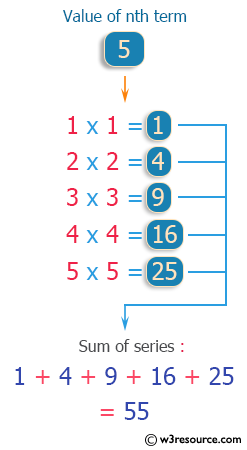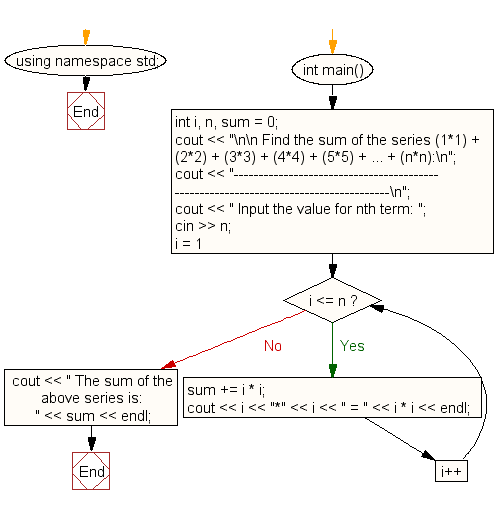﻿ C++ : Calculate the sum of the series (1*1)+(2*2)+.. +(n*n)

# C++ Exercises: Calculate the sum of the series (1*1) + (2*2) + (3*3) + (4*4) + (5*5) + ... + (n*n)

## C++ For Loop: Exercise-12 with Solution

Write a program in C++ to calculate the sum of the series (1*1) + (2*2) + (3*3) + (4*4) + (5*5) + ... + (n*n).

Pictorial Presentation:Sample Solution :-

C++ Code :

``````#include <iostream>
using namespace std;

int main()
{
int i, n, sum = 0;
cout << "\n\n Find the sum of the series (1*1) + (2*2) + (3*3) + (4*4) + (5*5) + ... + (n*n):\n";
cout << "------------------------------------------------------------------------------------\n";
cout << " Input the value for nth term: ";
cin >> n;

for (i = 1; i <= n; i++)
{
sum += i * i;
cout << i << "*" << i << " = " << i * i << endl;
}
cout << " The sum of the above series is: " << sum << endl;
}
``````

Sample Output:

``` Find the sum of the series (1*1) + (2*2) + (3*3) + (4*4) + (5*5) + ...
+ (n*n):
-----------------------------------------------------------------------
-------------
Input the value for nth term: 5
1*1 = 1
2*2 = 4
3*3 = 9
4*4 = 16
5*5 = 25
The sum of the above series is: 55
```

Flowchart:C++ Code Editor:

What is the difficulty level of this exercise?

﻿

## C++ Programming: Tips of the Day

Using C++ library in C code

You will need to write an interface layer in C++ that declares functions with extern "C":

```extern "C" int foo(char *bar)
{
returnrealFoo(std::string(bar));
}
```

Then, you will call foo() from your C module, which will pass the call on to the realFoo() function which is implemented in C++.

If you need to expose a full C++ class with data members and methods, then you may need to do more work than this simple function example.

Ref: https://bit.ly/3clHy43

We are closing our Disqus commenting system for some maintenanace issues. You may write to us at reach[at]yahoo[dot]com or visit us at Facebook